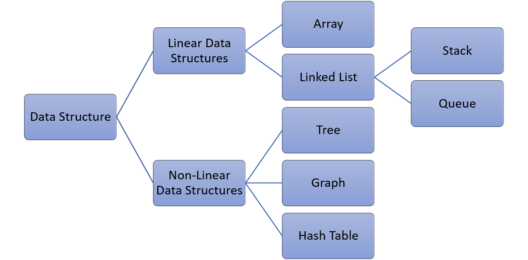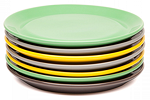# What are Stack Data Structures in Python?

Published on Aug 19,2019 167 ViewsData Structures are a collection of data values, the relationships among them, and the functions or operations that can be applied to the data. Now there are a lot of Data Structures available. But today our focus will be on Stack Data Structures. I’ll be discussing the following topics:

## Why Data Structures?

To answer this you will have to think on a big level. Think how Google maps show you the best route in just fraction of seconds, how it returns you search result in microseconds. It does not deal with only 100 websites, it deals with more than a billion sites and still shows you result so quickly.

Well, although the algorithm used plays a crucial role, the data structure or the container used is the foundation of that algorithm. In any application, organizing and storing data in a way or in a structure that is best suited to its usage is key to efficient access and processing of data.

## Types of Data Structures

There are some standard data structures that can be used to efficiently work with data. We can even customize them or build completely new ones to suit our application.## What is Stack Data Structure?

Consider some real-life examples:

• Shipment in cargo
• Plates on a tray
• Stack of coins
• Stack of drawers
• Shunting of trains in a railway yardAll of these examples follow a Last-In-First-Out strategy. Consider plates on a tray, When you want to pick a plate you are forced to pick a plate from the top whereas when the plates were kept on the tray they must be in reverse order. Above examples which follow the Last-In-First-Out (LIFO) principle are known as Stack.

Apart from the complementary operations, I may say that the main Operations possible on the stack are:

1. Push or insert an element to the top of the stack
2. Pop or remove an element from the top of the stack

## Creating Stack Data Structure

```class Stack:
def __init__(self,max_size):
self.__max_size=max_size
self.__elements=[None]*self.__max_size
self.__top=-1```

• max_size is the maximum number of elements expected in the stack.
• Elements of the stack are stored in the python list.
• Top indicates the topmost index of the stack which Is initially taken -1 to mark empty stack.

The initial status of the Stack can be seen in the Figure where max_size=5Push Element into Stack

Now, if you want to enter or push element to the stack, you have to remember that

• The top will point the index to which the element will be inserted.
• And that no element will be inserted when the stack is full i.e. when max_size=top.

So what should be the algorithm??

```# returns the maximum size of stack
def get_max_size(self):
return self.__max_size

# returns bool value whether stack is full or not, True if full and False otherwise
def is_full(self):
return self.get_max_size() - 1==self.__top

#pushes element at the top of stack
def push(self, data):
if(self.is_full()):
else:
self.__top=self.__top+int(1)
self.__elements[self.__top]=data

#You can use the below __str__() to print the elements of the DS object while debugging
def __str__(self):
msg=[]
index=self.__top
while(index>=0):
msg.append((str)(self.__elements[index]))
index-=1
msg=" ".join(msg)
msg="Stack data(Top to Bottom): "+msg
return msg```

Now, When you execute the following:

stack1=Stack(4)

#Push all the required element(s).

stack1.push(“A”)

stack1.push(“B”)

stack1.push(“C”)

stack1.push(“E”)

print(stack1.is_full())

print(stack1)Output:

True
Stack data(Top to Bottom): D C B A

## Pop Elements from Stack

Now, as you have inserted the elements into the stack, you want to pop them, so you need to take care of the following:

• Stack is not empty i.e. top != -1
• When you delete the data, the top must point to the previous top of the stack.

So, what will be the algorithm??

```#returns bool value whether stack Is empty or not, True if empty and False otherwise
def is_empty(self):
return self.__top==-1

#returns popped value
def pop(self):
if(self.is_empty()):
else:
a=self.__elements[self.__top]
self.__top=self.__top-1;
return a

#display all the stack elements from top to bottom
def display(self):
for i in range(self.__top,-1,-1):
print(self.__elements[i],end=' ')
print()```

Now, considering previously created stack, try to pop elements

print(stack1.pop())

print(stack1.pop())

print(stack1)

print(stack1.pop())

print(stack1.pop())

print(stack1.pop())Output:

D

C

Stack data(Top to Bottom): B A

B

A

## Applications of Stack Data Structure

• Example 1:

A stack is used to implementing bracket matching algorithm for arithmetic expression evaluation and also in the implementation of method calls.• Example 2:

Clipboard in Windows uses two stacks to implement undo-redo (ctrl+z, ctrl+y) operations. You would have worked on Windows word editors such as MS-Word, Notepad, etc. Here is a text is written in MS-Word. Observe how the text changed on click of Ctrl-Z and Ctrl-Y.Here is a code that simulates undo-redo operation. Go through the code and observe how the stack is used in this implementation.

```#creating class stack
class Stack:
def __init__(self,max_size):
self.__max_size=max_size
self.__elements=[None]*self.__max_size
self.__top=-1

def is_full(self):
if(self.__top==self.__max_size-1):
return True
return False

def is_empty(self):
if(self.__top==-1):
return True
return False

def push(self, data):
if(self.is_full()):
print("The stack is full!!")
else:
self.__top+=1
self.__elements[self.__top]=data

def pop(self):
if(self.is_empty()):
print("The stack is empty!!")
else:
data= self.__elements[self.__top]
self.__top-=1
return data

def display(self):
if(self.is_empty()):
print("The stack is empty")
else:
index=self.__top
while(index>=0):
print(self.__elements[index])
index-=1

def get_max_size(self):
return self.__max_size

#You can use the below __str__() to print the elements of the DS object while debugging
def __str__(self):
msg=[]
index=self.__top
while(index>=0):
msg.append((str)(self.__elements[index]))
index-=1
msg=" ".join(msg)
msg="Stack data(Top to Bottom): "+msg
return msg

#function to implement remove or backspace operation
def remove():
global clipboard,undo_stack
data=clipboard[len(clipboard)-1]
clipboard.remove(data)
undo_stack.push(data)
print("Remove:",clipboard)

#function to implement undo operation
def undo():
global clipboard,undo_stack,redo_stack
if(undo_stack.is_empty()):
print("There is no data to undo")
else:
data=undo_stack.pop()
clipboard.append(data)
redo_stack.push(data)
print("Undo:",clipboard)

#function to implement redo operation
def redo():
global clipboard, undo_stack,redo_stack
if(redo_stack.is_empty()):
print("There is no data to redo")
else:
data=redo_stack.pop()
if(data not in clipboard):
print("There is no data to redo")
redo_stack.push(data)
else:
clipboard.remove(data)
undo_stack.push(data)
print("Redo:",clipboard)

clipboard=["A","B","C","D","E","F"]
undo_stack=Stack(len(clipboard))
redo_stack=Stack(len(clipboard))
remove()
undo()
redo()```

Output:

Remove: [‘A’, ‘B’, ‘C’, ‘D’, ‘E’]

Undo: [‘A’, ‘B’, ‘C’, ‘D’, ‘E’, ‘F’]

Redo: [‘A’, ‘B’, ‘C’, ‘D’, ‘E’]

With this, we come to an end of this Stack Data Structure in Python article. If you successfully understood and ran the codes by yourselves you are no longer a newbie to Stacks Data Structure.

Got a question for us? Please mention it in the comments section of this article and we will get back to you as soon as possible.

To get in-depth knowledge on Python along with its various applications, you can enroll for live Python online training with 24/7 support and lifetime access.REGISTER FOR FREE WEBINARThank you for registering Join Edureka Meetup community for 100+ Free Webinars each month